# Binary Subtractor

Before discussing about binary substractor, let us discuss about method of substracting two multi bit binary numbers.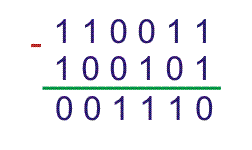For above substraction we used general rules which are,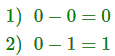and borrow 1 which to be added to next higher significant bit of first binary number. Then same positioned bit of second binary number would be substracted from that.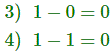But there are other methods by which two binary numbers can be substracted confidently. One of these is 2’s complement method of substraction.

Here, first binary number (from which another binary number to be substracted) is kept as it is. Then each bit of second binary numbers (which to be substracted) is complemented. Then 1 is added to LSB of complemented second binary number. This results 2’s complement of second binary number. Now finally we add first binary number with 2’s complement of the second binary number and we get final result of substraction.
Here in the previous example,
First binary number was 110011 and second binary number was 100101. Complement or 1’s complement of 100101 is 011010. Now by adding 1 with LSB of this 1’s complement number we get,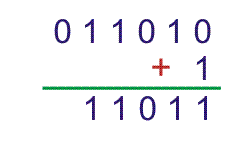Now by adding first number, 110011 and 2’s complement of second number i.e. 11011. We get,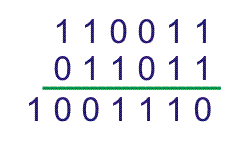Hence, 4 bit substractor can be drawn like,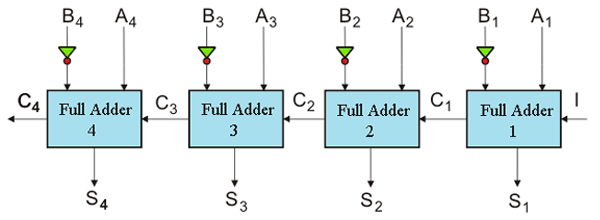Here, A4, A3, A2, A1 is minuend and B4, B3, B2, B1 is subtrahend. S4, S3, S2, S1 is result of substraction where C4 is final carry which is ignored.

## Half Substractor

Half substractor is a combinational circuit which performs substraction of single bit binary numbers. The substraction combinations of two single bit binary numbers can be,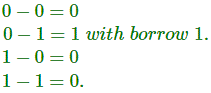Now if we draw a truth table for that, with all differences (D) and borrow (b), we get,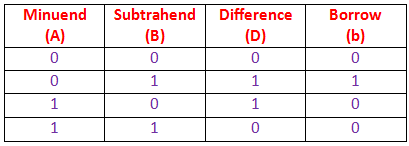Hence, from truth table it is found that,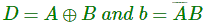The above equations can be represented using logic gates.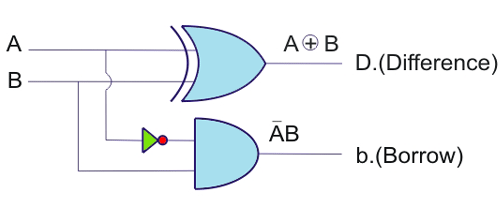The above circuit is logical half substractor circuit.

## Full Substractor

This is not practical to perform substraction only between two single bit binary numbers. Instead binary numbers are always multibits. The substraction of two binary numbers is performed bit by bit from right (LSB) to left (MSB). During substraction of same significant bit of minuend and subtrahend, there may be one borrow bit along with difference bit. This borrow bit (either 0 or 1) is to be added to the next higher significant bit of minuend and then next corresponding bit of subtrahend to be subtracted from this. It will continue up to MSB. The combinational logic circuit performs this operation is called full substractor. Hence, full substractor is similar to half substractor but inputs in full substractor are three instead of two.
Two inputs are for the minuend and subtrahend bits and third input is for borrowed which comes from previous bits substraction. The outputs of full adder are similar to that of half adder, these are difference (D) and borrow (b).

The combination of minuend bit (A), subtrahend bit (B) and input borrow (bi) and their respective differences (D) and output borrows (b) are represented in a truth table, as follow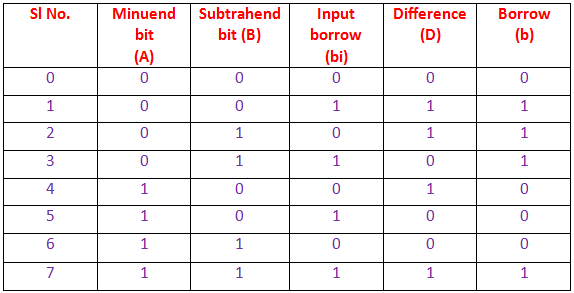Let us draw K-map for D and b.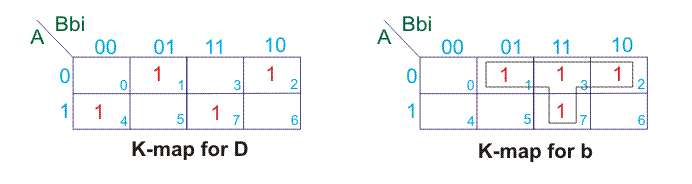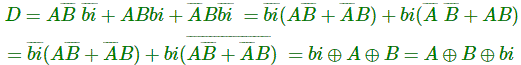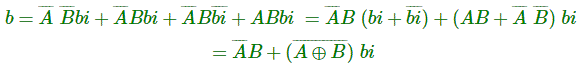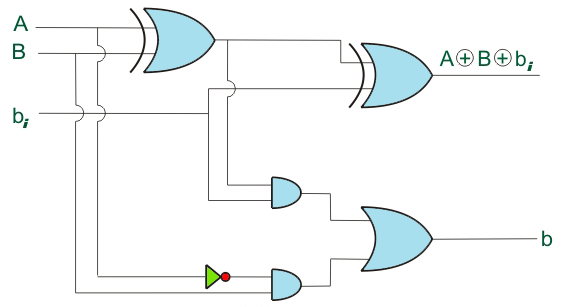We have already designed 4 bits binary parallel adder and 4 bit binary substractor. We have also seen that both circuits are more or less same except in substractor the subtrahend bit inputs are inverted with input borrow bit at LSB is 1.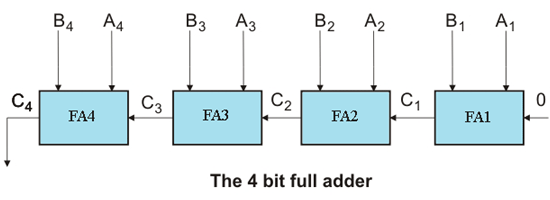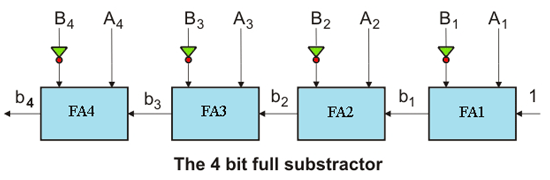In the above 4 bit full adder circuit, third input to LSB Adder (FAI) is 1. In addition to that, in full substractor substandent bits, i.e. B1, B2, B3 and B4 are inverted.
We can combine these two circuits (Adder and Substractor) in one circuit by controlling B1, B2, B3 and B4 terminals and third input of LSB adder unit (FAI). We know that,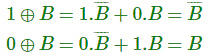So, we can use XOR gate at each input B1, B2, B3 and B4 with control input M (either 1 or 0).
Now, if M = 1, B1, B2, B3 and B4 will be complemented. At the same time if third input of FAI is 1, the circuit becomes substractor. So, M = 1 is also to be fed to the third input of FAI in substractor.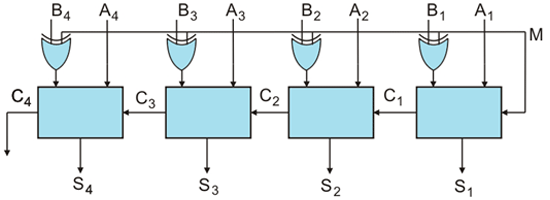Want To Learn Faster? 🎓
Get electrical articles delivered to your inbox every week.
No credit card required—it’s 100% free.